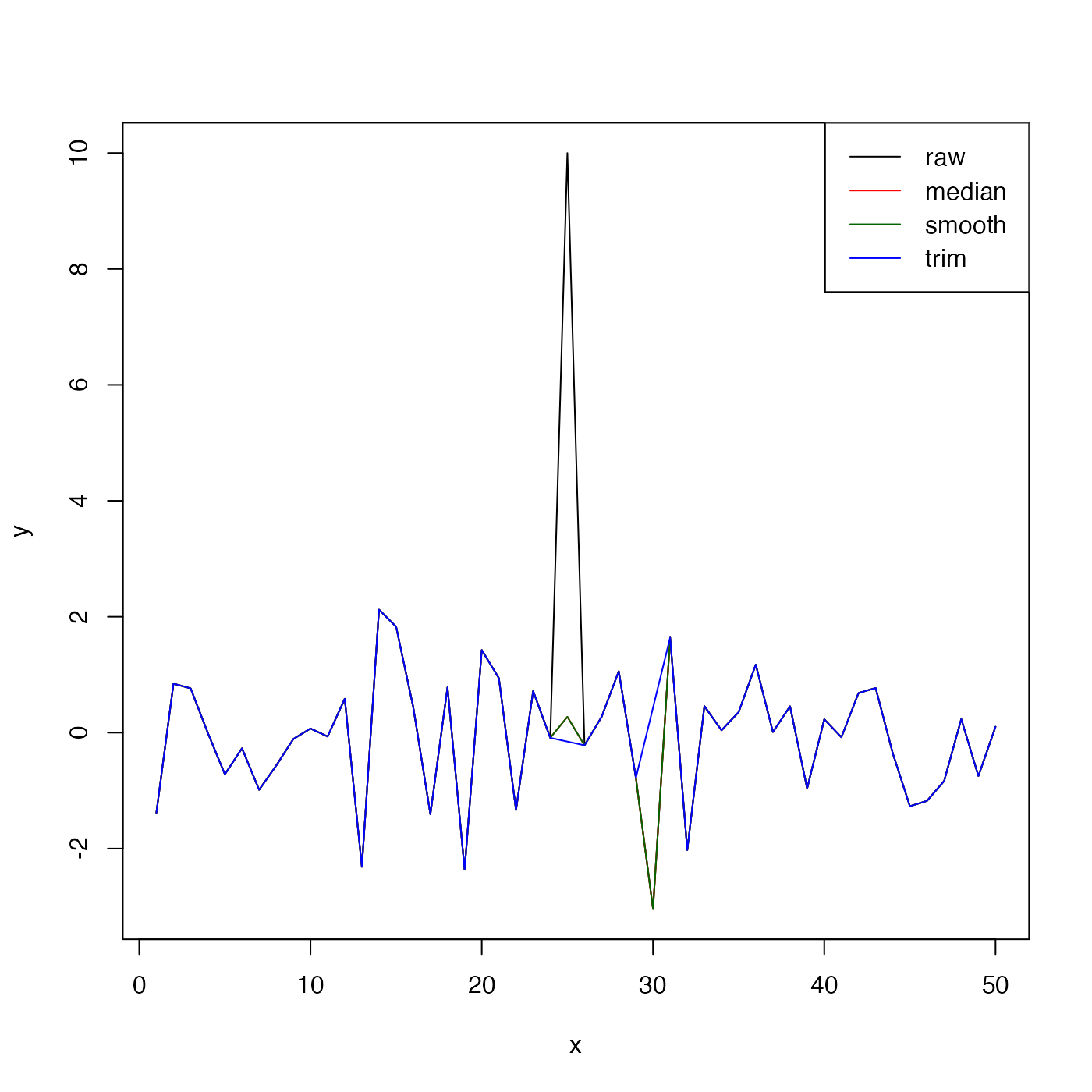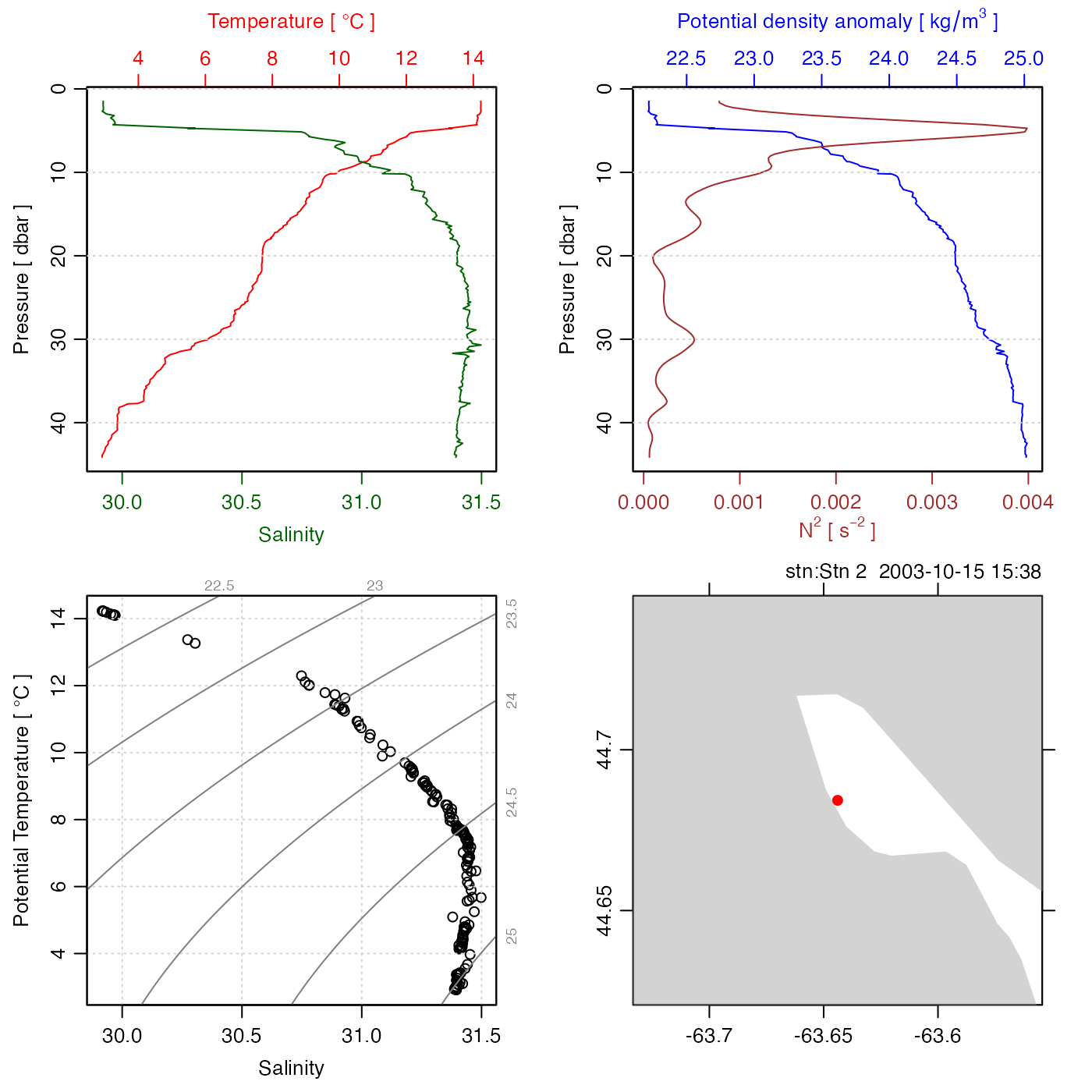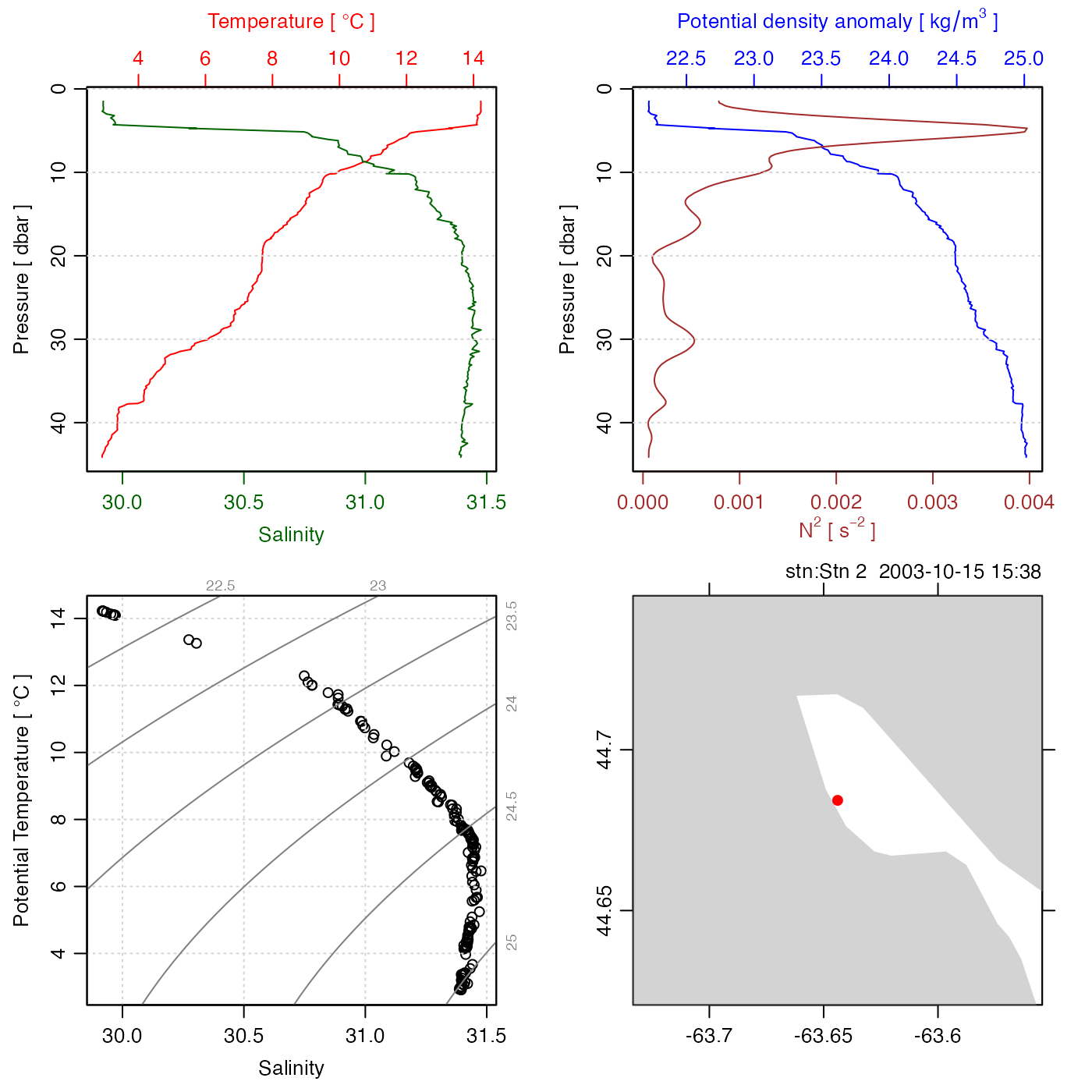The method identifies spikes with respect to a "reference" time-series, and replaces these spikes with the reference value, or with NA according to the value of action; see “Details”.

despike(
x,
reference = c("median", "smooth", "trim"),
n = 4,
k = 7,
min = NA,
max = NA,
replace = c("reference", "NA"),
skip
)

## Arguments

x a vector of (time-series) values, a list of vectors, a data frame, or an oce object. indication of the type of reference time series to be used in the detection of spikes; see ‘Details’. an indication of the limit to differences between x and the reference time series, used for reference="median" or reference="smooth"; see ‘Details.’ length of running median used with reference="median", and ignored for other values of reference. minimum non-spike value of x, used with reference="trim". maximum non-spike value of x, used with reference="trim". an indication of what to do with spike values, with "reference" indicating to replace them with the reference time series, and "NA" indicating to replace them with NA. optional vector naming columns to be skipped. This is ignored if x is a simple vector. Any items named in skip will be passed through to the return value without modification. In some cases, despike will set up reasonable defaults for skip, e.g. for a ctd object, skip will be set to c("time", "scan", "pressure") if it is not supplied as an argument.

## Value

A new vector in which spikes are replaced as described above.

## Details

Three modes of operation are permitted, depending on the value of reference.

1. For reference="median", the first step is to linearly interpolate across any gaps (spots where x==NA), using approx() with rule=2. The second step is to pass this through runmed() to get a running median spanning k elements. The result of these two steps is the "reference" time-series. Then, the standard deviation of the difference between x and the reference is calculated. Any x values that differ from the reference by more than n times this standard deviation are considered to be spikes. If replace="reference", the spike values are replaced with the reference, and the resultant time series is returned. If replace="NA", the spikes are replaced with NA, and that result is returned.

2. For reference="smooth", the processing is the same as for "median", except that smooth() is used to calculate the reference time series.

3. For reference="trim", the reference time series is constructed by linear interpolation across any regions in which x<min or x>max. (Again, this is done with approx() with rule=2.) In this case, the value of n is ignored, and the return value is the same as x, except that spikes are replaced with the reference series (if replace="reference" or with NA, if replace="NA".

## Examples

n <- 50
x <- 1:n
y <- rnorm(n=n)
y[n/2] <- 10                    # 10 standard deviations
plot(x, y, type='l')lines(x, despike(y), col='red')lines(x, despike(y, reference="smooth"), col='darkgreen')lines(x, despike(y, reference="trim", min=-3, max=3), col='blue')legend("topright", lwd=1, col=c("black", "red", "darkgreen", "blue"),
legend=c("raw", "median", "smooth", "trim"))# add a spike to a CTD object
data(ctd)
plot(ctd)T <- ctd[["temperature"]]
T <- T + 10
ctd[["temperature"]] <- T
CTD <- despike(ctd)
plot(CTD)